【本文参与征文活动】

2020.11.11更新

## 基础语法部分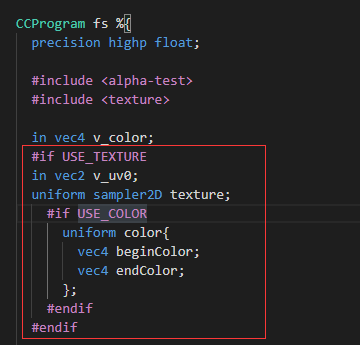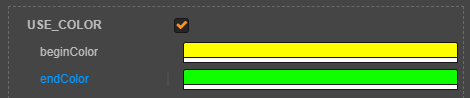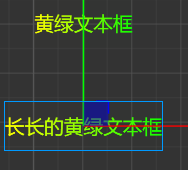tips

1. #if 用于编辑器内那些框框的显示，如果是代码逻辑 使用if
2.in 传入的变量 不可被修改 out 传出的变量，如下所示，不过我搞不懂为什么顶点着色器能拿到a_uv0这个值,下面的片元着色器就拿不到了，希望有大佬解惑。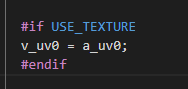3.不能传入float,vec4之类的变量，需要把它加到一个模块中，这个我觉得是我的写法有问题，求大佬解惑。4.编辑器与浏览器显示效果不一致时，一般是合图导致的，这时只需关掉图片的合图即可。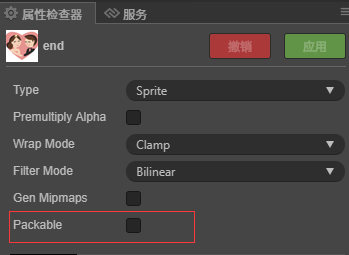## 文本部分

`a_color = beginColor + (endColor-beginColor)*uv.y;`
`a_color = beginColor + (endColor-beginColor)*(uv.x+uv.y)/2.0``uv.y = uv.y + 0.2 *sin( 3.14 * uv.x); `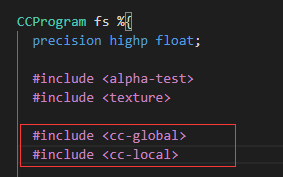`uv.y = uv.y + 0.2 *sin( 3.14 * uv.x)*sin(7.77*cc_time.x);`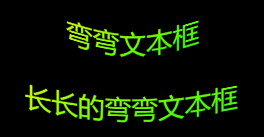` a_color = beginColor + (endColor-beginColor)*( uv.x + sin(7.77*cc_time.x)) ;`` if( abs( tan(cc_time.x) - (uv.x+uv.y)/2.0 ) <0.05 ) { a_color = endColor ; }`

` // if( abs( sin(cc_time.x) - (uv.x+uv.y)/2.0 ) <0.05 ) { // a_color = endColor ; // }else if(abs( sin(cc_time.x) - (1.0-uv.x+uv.y)/2.0 ) <0.05){ // a_color = beginColor ; // }`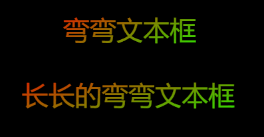## 图片部分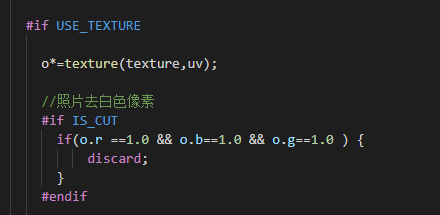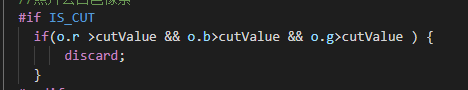` //流光效果 #if IS_FLUXAY if( abs( tan(cc_time.x) - (uv.x+uv.y)/2.0 ) <0.02 ) { a_color = fluaxyColor; } #endif`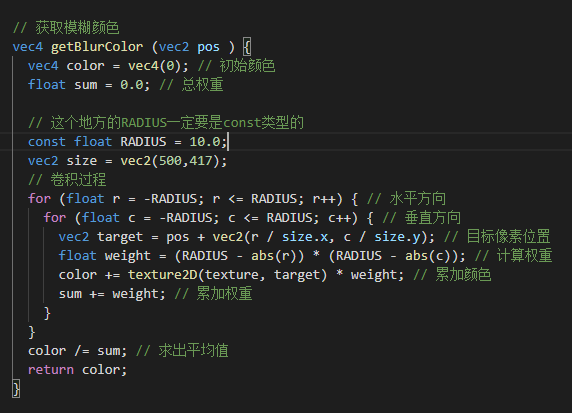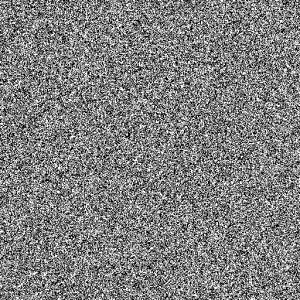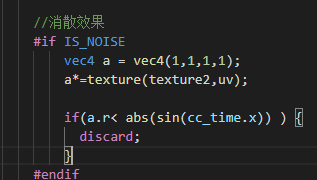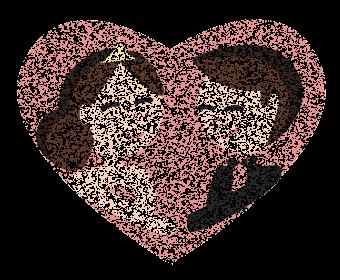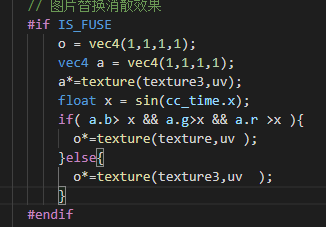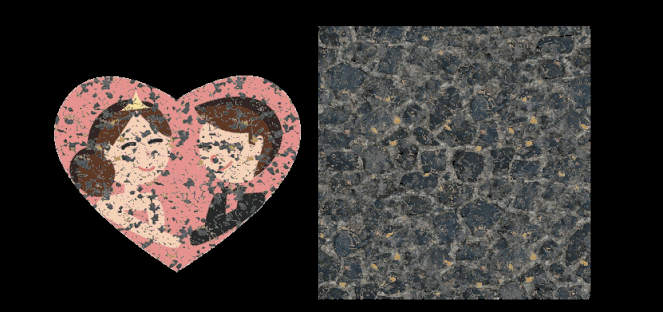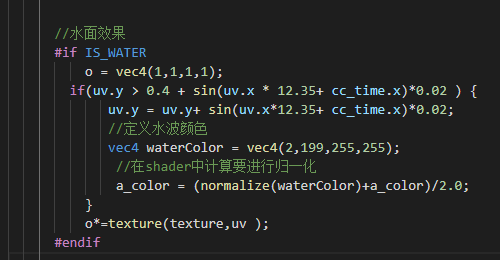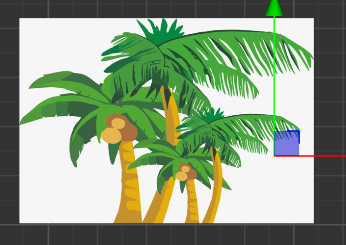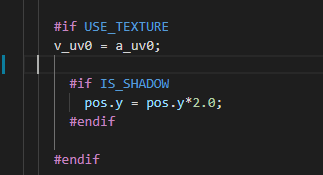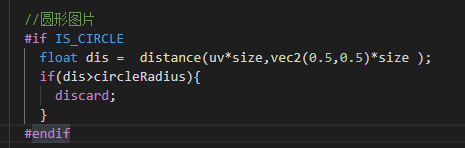24赞

mark 学习mark.